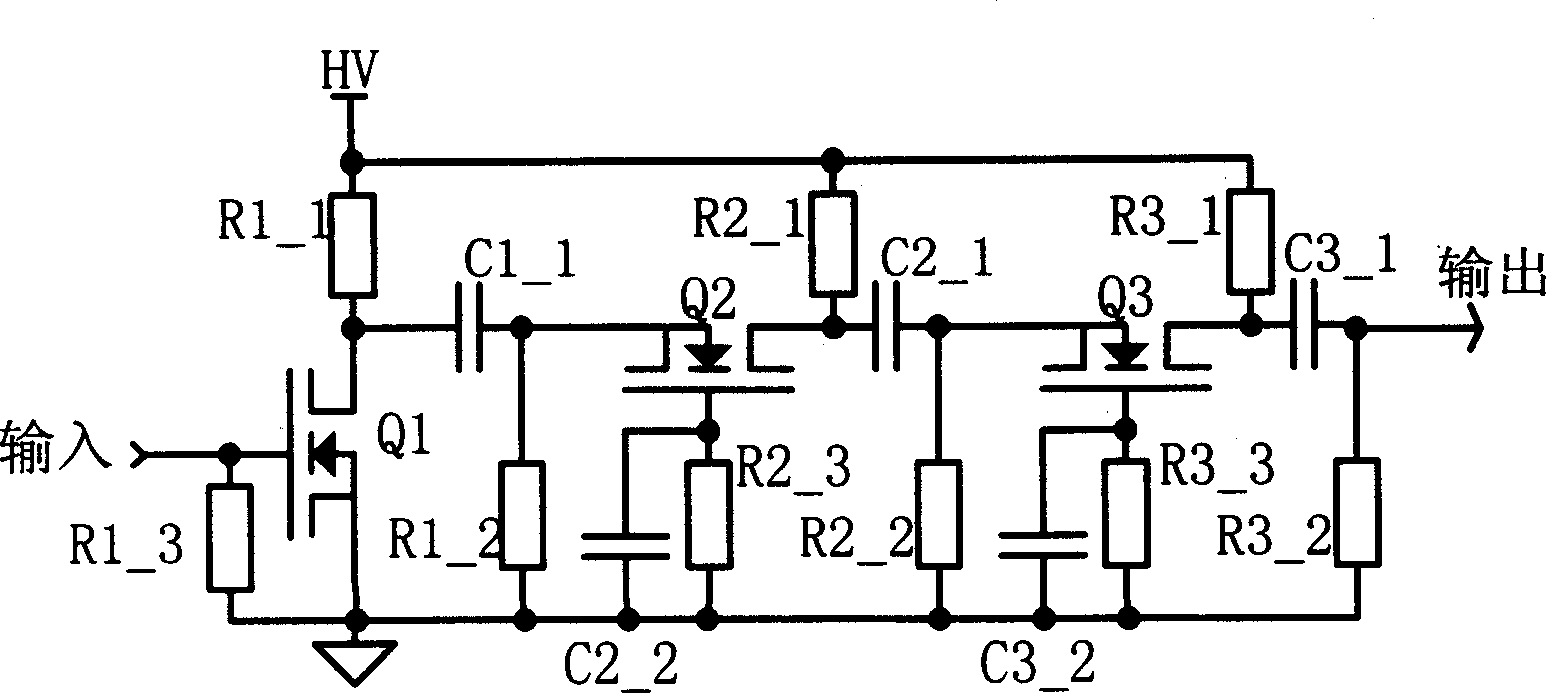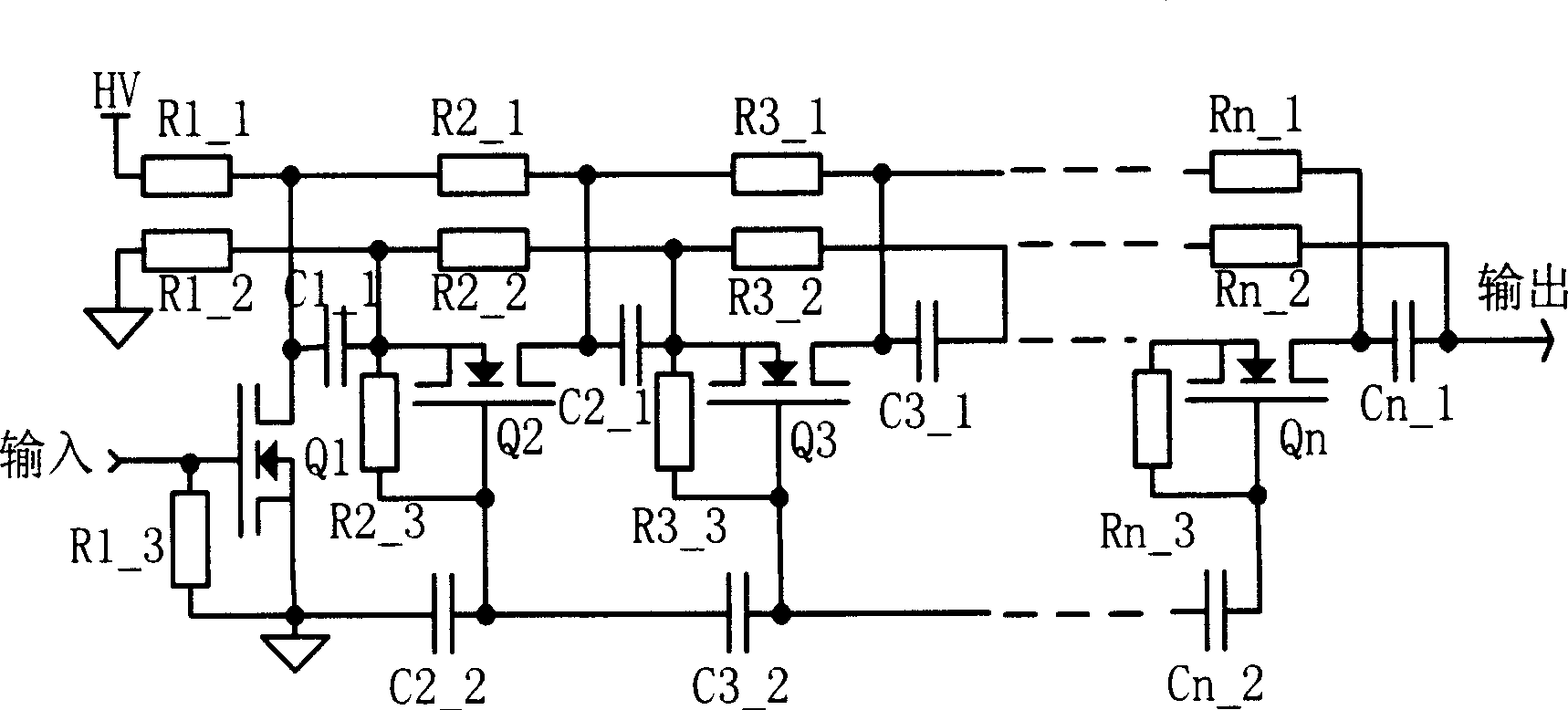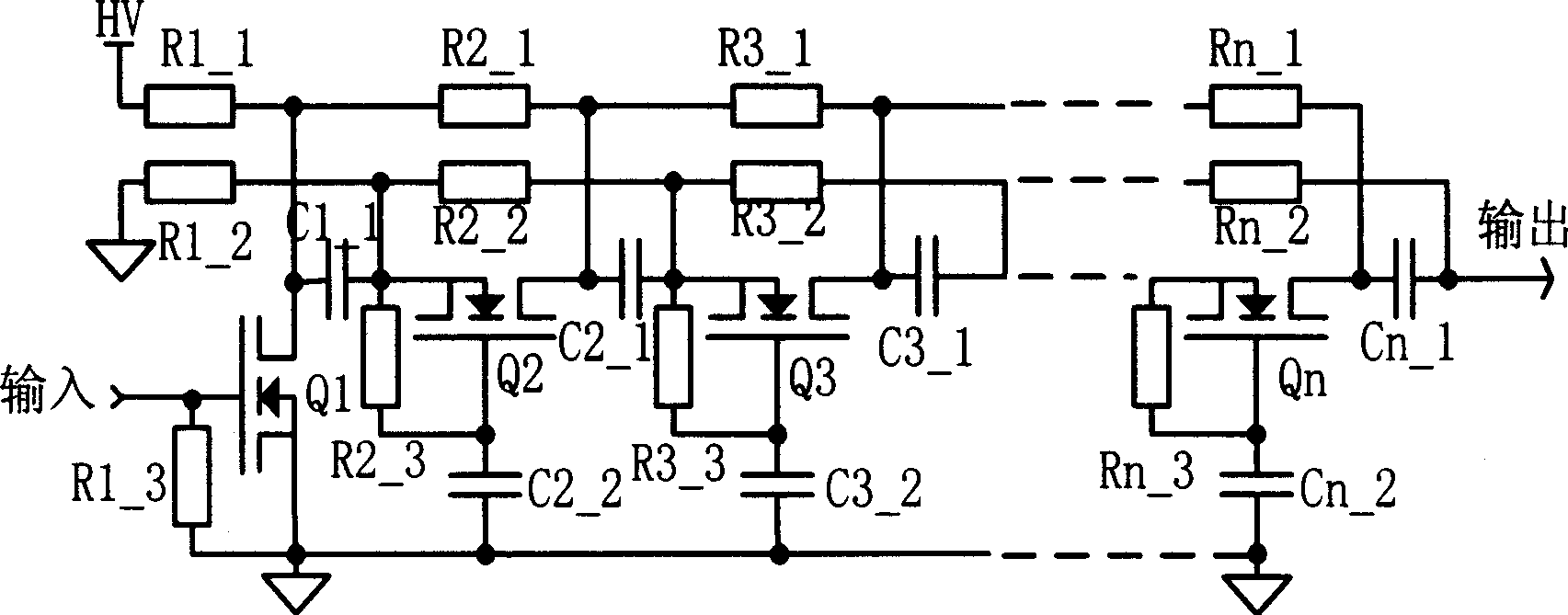# High speed high voltage switch circuit

## A switching circuit and high-voltage technology, which is applied in the field of high-voltage and high-speed pulses, can solve the problems of difficulty, high-voltage switches, large volume, and high cost

Inactive Publication Date: 2006-06-28
SHANGHAI INST OF OPTICS & FINE MECHANICS CHINESE ACAD OF SCI
0 Cites 2 Cited by

## AI-Extracted Technical Summary

### Problems solved by technology

At present, there are also switching circuits based on field effect transistors (MOSFETs), such as figure 1 In the voltage doubler switch circuit shown, when the input trigger signal is turned on, the closer to the output terminal, the higher the voltag...
View more

## Abstract

A reliable serial double-voltage high speed and high voltage switch circuit is based on the connection of multi-stage field effect transistors including: a first stage composed of the field effect transistor, three resistors and a condenser, the second stage to the nth stage composed of an nth field effect transistor, an n1 resistor, an n2 resistor, an n3 resistor, an n1 condenser and an n2 condenser, which puts a low demand on the elements and outputs high amplitude pulse voltages by multiple stages of double voltages.

Application Domain

Electronic switching

Technology Topic

Pulse voltageLow demand +9

## Image

•••## Examples

• Experimental program(1)

### Example Embodiment

 The present invention will be described in detail below in conjunction with the accompanying drawings and embodiments.
 see first figure 2 , figure 2 It is the circuit diagram of Embodiment 1 of the high-speed high-voltage switch circuit of the present invention. It can be seen from the figure that the high-speed high-voltage switch of the present invention is composed of multiple stages in series, and each stage is composed of field effect transistors, capacitors, and resistors. Its composition includes:
 The first stage is composed of the first field effect transistor Q1, the eleventh resistor R1_1, the twelfth resistor R1_2, the thirteenth resistor R1_3, and the eleventh capacitor C1_1;
 The second stage is composed of the second field effect transistor Q2, the 21st resistor R2_1, the 22nd resistor R2_2, the 23rd resistor R2_3, the 21st capacitor C2_1, and the 22nd capacitor C2_2;
 The structure of the third stage to the nth stage is the same as that of the second stage, that is, the nth stage is composed of the nth field effect transistor Qn, the n1st resistor Rn_1, the n2th resistor Rn_2, the n3rd resistor Rn_3, the n1st capacitor Cn_1, and the n2th capacitor The composition of Cn_2, the specific connection relationship of components at all levels:
 Stage 1: The gate of the first FET Q1 is connected to one end of the thirteenth resistor R1_3 and the input terminal of the input signal, and the other end of the thirteenth resistor R1_3 is grounded; the source of the first FET Q1 is grounded; 1 The drain of the field effect transistor Q1 is connected to one end of the 11th capacitor C1_1, one end of the 11th resistor R1_1 and one end of the 21st resistor R2_1 of the second stage, and the other end of the 11th capacitor C1_1 is connected to one end of the 12th resistor R1_2 And connected to the source of the second field effect transistor Q2 of the second stage, the other end of the twelfth resistor R1_2 is grounded, and the other end of the eleventh resistor R1_1 is connected to the high voltage input terminal HV;
 Stage 2: The gate of the second field effect transistor Q2 is connected to one end of the 23rd resistor R2_3, one end of the 22nd capacitor C2_2, and one end of the 32nd capacitor C3_2 in the third stage, and the other end of the 22nd capacitor C2_2 is grounded; 2. The source of the field effect transistor Q2 is connected to the other end of the 23rd resistor R2_3, one end of the 22nd resistor R2_2 and connected to the 11th capacitor C1_1 of the previous stage and one end of the 12th resistor R1_2, and the other end of the 22nd resistor R2_2 is connected to the 22nd resistor R2_2 One end of the 21st capacitor C2_1 is connected to the source of the third field effect transistor Q3 in the third stage; the drain of the second field effect transistor Q2 is connected to one end of the 21st resistor R2_1 and the other end of the 21st capacitor C2_1 and connected to the other end of the 21st capacitor C2_1 One end of the 31st resistor R3_1 of the third stage is connected, and the other end of the 21st resistor R2_1 is connected to the drain of the first field effect transistor Q1 of the first stage;
...;
 The i-th stage: the gate of the i-th field effect transistor Qi is connected to one end of the i3-th resistor Ri_3, one end of the i2-th capacitor Ci_2, and one end of the i+1-th capacitor Ci+1_2 in the next stage, and the other end of the i2-th capacitor Ci_2 It is connected to the grid of the i-1th field effect transistor Qi-1 of the previous stage; the source of the i-th field effect transistor Qi is connected to the other end of the i3th resistor Ri_3 and one end of the i2th resistor Ri_2 and is connected to the front stage (i -1) 1 capacitor Ci-1_1, one end of the (i-1) 2nd resistor Ri-1_2 is connected, the other end of the i2th resistor Ri_2 is connected to one end of the i1th capacitor Ci_1 and connected to the i+1th field of the next stage The source of the effect transistor Qi+1 is connected; the drain of the i-th field effect transistor Qi is connected to one end of the i1-th resistor Ri_1, the other end of the i1-th capacitor Ci_1, and connected to the i+1-th resistor Ri+1_1 of the next stage One end is connected, and the other end of the i1th resistor Ri_1 is connected to the drain of the i-1th field effect transistor Qi-1 of the previous stage;
...;
 The connection relationship of the components of the last nth stage: the gate of the nth field effect transistor Qn is connected to one end of the n3th resistor Rn_3 and one end of the n2th capacitor Cn_2, and the other end of the n2th capacitor Cn_2 is connected to the n-1th field of the previous stage The gate of the effect transistor Qn-1 is connected; the source of the nth field effect transistor Qn is connected to the other end of the n3th resistor Rn_3, one end of the n2th resistor Rn_2 and connected to the (n-1)1st capacitor Cn-1_1 of the previous stage 1. One end of the (n-1) 2nd resistor Rn-1_2 is connected, and the other end of the n2th resistor Rn_2 is connected with one end of the n1st capacitor Cn_1 to form the output end of the switch circuit; the drain of the nth field effect transistor Qn is connected to the One end of the n1th resistor Rn_1 is connected to the other end of the n1th capacitor Cn_1, and the other end of the n1th resistor Rn_1 is connected to the drain of the n-1th field effect transistor Qn-1 of the previous stage.
 Below to figure 2 The circuit is taken as an example to illustrate the working principle of the present invention. When the input pulse triggers the first field effect transistor Q1, the first field effect transistor Q1 is quickly turned on, and the drain potential of the first field effect transistor Q1 quickly becomes zero, so at the other end of the eleventh capacitor C1_1, that is, at the 2 The source S of the FET Q2 builds up a negative high voltage with an amplitude of HV, and part of the charge on the 11th capacitor C1_1 is quickly distributed to the 22nd capacitor C2_2 and the gate-source equivalent capacitance of the 2nd FET Q2 On CgsEIF, when the capacitance of the 22nd capacitor C2_2 is appropriate and the voltage established on CgsEIF is greater than the threshold voltage of the second field effect transistor Q2, the second field effect transistor Q2 is also quickly turned on, so in the third field The source of the effect transistor Q3 establishes a negative high voltage with an amplitude of 2HV, and so on until the output terminal of the nth stage outputs a negative pulse of nHV. The resistors Rn_1, Rn_2, and Rn_3 of each stage provide a static operating point for the field effect transistor Qn of this stage and form a charge charging and discharging circuit. When the circuit parameters are properly matched, at the moment when the input pulse signal is triggered, all field effect transistors are turned on almost simultaneously, and a nanosecond-level high-voltage pulse is obtained at the output end. The high-voltage switching circuit mainly controls the gate-source voltage of MOSFETs at all levels, unlike the switching circuit composed of avalanche tubes, which mainly controls the avalanche bias voltage of each transistor, so the adjustment range of the output high-voltage pulse is larger than that composed of avalanche tubes. High voltage switches have a much wider range of adjustment.
 Through analysis we know that in figure 1 In the existing circuit shown, the closer to the output stage, the greater the voltage that the bias resistor and the voltage dividing capacitor between the gate and the ground will withstand, so use figure 1 The high-voltage switch composed of the circuit shown has high requirements on the withstand voltage performance of components and components, and the volume is large. That is to say, due to the limitation of the performance of components and components, the number of voltage multiplication stages cannot be many, and the output pulse high voltage is not easy to be too high. No matter the high-voltage switch constituted by the present invention is static (the tube is not turned on) or the instant of conduction, the potential difference borne by all the components does not exceed the bias voltage HV, it has the characteristics of small size, low cost, etc., and can be connected in many stages Together, a very high output pulse voltage is obtained.
 If the circuit has only one stage, output pulse high voltage from the end connecting the 11th capacitor C1_1 to the 12th resistor R1_2; if the circuit has two stages, output pulse high voltage from the end connecting the 21st capacitor C2_1 to the 22nd resistor R2_2; if the circuit has many stage, the pulse high voltage is output from the end connected to the n1th capacitor Cn_1 and the n2th resistor Rn_2.
 In the case of a small number of circuit stages, one end of the capacitors C3_2 , C4_2 up to the capacitor Cn_2 of the last stage connected to the field effect transistor of the previous stage can be grounded. Specific circuit such as image 3 as shown, image 3 It is the circuit diagram of Embodiment 2 of the high-speed high-voltage switching circuit of the present invention. The second capacitor (C3_2, C4_2...Cn_2) of the third stage to the nth stage described in the figure is directly grounded with one end connected to the previous stage field effect transistor. figure 2 The difference of Embodiment 1, in other words, is only that the capacitance Cn_2 connected between the gates of each stage is grounded in series instead of grounded separately. The number of stages is mainly determined by the capacitance, rated withstand voltage and volume of the capacitor Cn_2. Since the n2th capacitor Cn_2 is generally in the order of picofarads, and the capacitor with a breakdown voltage in the order of kilovolts is not large, direct grounding can reduce the mutual influence between stages and facilitate adjustment.

## PUM## Description & Claims & Application Information

We can also present the details of the Description, Claims and Application information to help users get a comprehensive understanding of the technical details of the patent, such as background art, summary of invention, brief description of drawings, description of embodiments, and other original content. On the other hand, users can also determine the specific scope of protection of the technology through the list of claims; as well as understand the changes in the life cycle of the technology with the presentation of the patent timeline. Login to view more.
Who we serve
• R&D Engineer
• R&D Manager
• IP Professional
Why Eureka